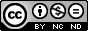## Sunday, May 11, 2014

### Portfolio Management - A Financial Approach - Part 3

Introduction
In the first post of this series we introduced some statistical concepts, useful to analyze and optimize the composition of a portfolio of projects. In particular, we have characterized projects as black boxes, which absorb resources and generate returns.
In the second post of this series we have derived the concept and mathematical formulation of what is called the efficient frontier, for a simple portfolio made up of two projects.
In this post we will explore a little more in depth the meaning of the the efficient frontier, analyzing its utility and how to use it to manage portfolio in a more effective way.
For sake of simplicity, we will stick to the hypothesis of  a simple 2 projects portfolio a little while yet.

The efficient frontier - What is this function telling us?
In Figure 1 we can see an example of efficient frontier for a 2 project portfolio. The reference equations are derived and explained in the second post of this series.

Each point of the curve depicted in Figure 1 represents a particular split of the portfolio's budget, that is, the percentage of budget invested in project 1 and in project 2. The efficient frontier assigns to each budget subdivision and hence to each portfolio's composition, values of average return and return’s uncertainty.

It is therefore possible to decide a budget subdivision between the 2 projects and check the so obtained portfolio's average return and standard deviation.
The other way round is also possible, we can choose a budget subdivision between the two projects to get a desiderable  average return, trying to remain inside defined range of return’s standard deviation.

A fundamental characteristic of the efficient frontier is that there is no better possible budget allocation, in the sense that, considering the projects at hand, we could not obtain a portfolio with a higher average return and a lower or equal standard deviation not residing on the frontier itself.Figure 2.

On what part of the efficient frontier we would like our portfolio to be?
In Figure 2 we have split the efficient frontier in three parts. Remember that each point of the efficient frontier is a budget subdivision among the projects that compose the portfolio, to which are associated an overall average return and a return’s standard deviation.
• Red zone (A - B) Whatever happens, it is important to try to avoid this zone. As it is clearly depicted in Figure 1 and Figure 2, it is possible to find points on the Orange zone or Green zone with a greater average return and an equal return’s standard deviation. That is to say, there are ways to split the budget among projects that can lead to a greater average return with the same uncertainty.
• Orange zone (B - C) In this zone it is easy to see that the average return grows faster than the associated standard deviation. That is to say, moving from B toward C, we could achieve increases for the average returns that are greater than the increases of the associated uncertainty. So, even if it is not possible to find budget's subdivisions that lead to more efficient portfolio composition (greater average returns with equal or smaller uncertainty), it could be a good idea move toward the Green zone. A mathematical way to view the same concept is to find the place where the first derivative of the efficient frontier with respect to the return’s standard deviation gets smaller than 1.
• Green zone (C - D) This is the better zone of the efficient frontier and possibly where a portfolio should be placed.

The effect of covariance on the efficient frontier
The covariance of the projects that compose the portfolio have an important effect on the efficient frontier's shape and position, as we can see In Figure 3. In the example the covariance between the 2 projects varies from 0 to +5 with unitary steps. The more the covariance increases, the more the efficient frontier moves towards the right of the axes. That is to say, we have to accept bigger risks to get the same average return. This is correct, because both the projects tend to go bad or well together. This leaves the average return more or less unchanged but it affects the associated uncertainty, spreading its magnitude.Figure 3.

So it is clear that if we want to build a coherent and harmonized portfolio, the projects' covariances can be a very important resource, since they can be appropriately mixed to modulate the uncertainty associated to the portfolio's average return.

Where can we stay on the efficient frontier?
The theory here explained, had been originally developed to analyze and manage securities portfolios, where every budget partition is theoretically allowed. This leads to the fact that the efficient frontier is often represented as a continuous function and that should be possible to place a portfolio on each one of its points. On the contrary, projects' investments are often quantized. This constrains a projects portfolio to stay on just some points of the efficient frontier.
As an example, we could diminsh the investment in a project from 120000 to 100000 dollars changing the quality requirements of some deliverables, but there could be no way to invest less than 100000 dollars or an amount of money between 100000 and 120000 dollars.Figure 4.

In Figure 4 we can see how a quantized efficient frontier looks like. In this case we could be able to place our portfolio just on the coloured dots. That could be seen as a limitation right now but it will help us later, when we will remove the 2 projects hypothesis.

In the next post we will remove the 2 projects hypothesis and we will apply what we have seen until now to a generic N-projects portfolio.Quest' opera è distribuita con licenza Creative Commons Attribuzione - Non commerciale - Non opere derivate 3.0 Unported.